The Theory \ Details \ parameters estimation \ advanced estimates

# Model Parameters Estimation

### Stambaugh combined-sample estimates for means and covariances

This technique consists in obtaining maximum-likelihood estimates of the model parameters for assets with different start dates and common final date.

In the event of portfolio components having different depths of historical data standard parameters estimates, considered in the previous section, result in a loss of information. The covariance matrix of portfolio components, calculated based on a historical dataset with different start dates is not necessarily positive-definite. Hence, the analyzed dataset is truncated traditionally to the date corresponding to the asset with the shortest history. With such an approach, the loss of the information contained in the initial dataset set is inevitable.

The method suggested in
[Stambaugh; 1997], consists in the consecutive use of the regression of assets with a shorter history on assets with a longer history. This time all the information contained in the data is used.

Note. It is worth mentioning that the use of the entire dataset improves estimates for longer-history assets as well as estimates for shorter-history assets.

For more detailed information on the discussed method see
[Stambaugh; 1997].

The shrinkage estimator is a statistical tradeoff between the bias and the estimation error. For the first time the shrinkage estimator appeared in [Stein; 1956], where it was shown that “shrinking” sample means of multivariate normal distribution to an appropriate common constant improves estimation accuracy.

In general, the shrinkage estimate is obtained via a “shrinkage” of sample unbiased estimate towards some biased target with lower estimation error.

Shrinking sample excess Mu towards excess Mu of GMV portfolio
Shrinking sample covariance matrix towards constant correlations covariance matrix
Shrinking sample estimates towards values, implied by asset pricing model

### Shrinking sample excess Mu towards excess Mu of GMV portfolio

Shrinkage estimator for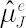was obtained in [Jorion; 1986]. Based on Bayes approach, estimateimplies shrinking os sample Mu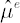towards a common constant, equal to sample excess Mu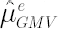for global minimum variance portfolio. Calculation formulae forare presented below: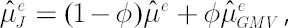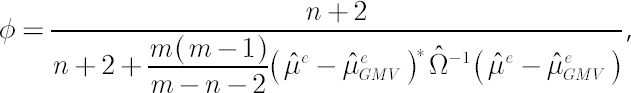whereis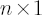vector of ones;is number of assets;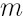is number of observations.

### Shrinking sample covariance matrix towards constant correlations covariance matrix

This estimate is a shrinkage estimator for covariance matrix. It was proposed in [Ledoit, Wolf; 2004].
Final estimate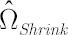is obtained via shrinkage of the sample covariance matrix towards the covariance matrix, produced by averaging correlations across asset pairs.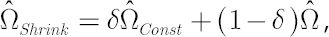where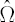– sample covariance matrix,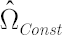– constant correlations covariance matrix.

Details, including the formulas for the optimal shrinkage intensity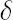can be found in
[Ledoit, Wolf; 2004].

### Shrinking sample estimates towards values, implied by asset pricing model

The given method of the estimation of parameters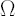and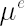, first suggested in [Pastor, Stambaugh; 1999], consist in applying Bayes approach, which assumes a certain degree of investor's confidence in that the market satisfies the selected factor-based asset pricing model (such as CAPM or Fama-French 3-factor model).

The degree of investor’s confidence in the chosen factor model is determined by the parameter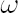, taking values in the range form 0 to 1. Value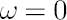means that the investor completely ignores predictions of the factor model, using as estimates forandtheir sample counterparts, while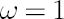means, that the estimates forandcoincide with
model-implied estimatesand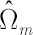.

Analytical formulas in general caseare presented in
[Wang; 2005].

### Joint estimator for means and covariances based on Missing Factor approach

This method described in [MacKinlay, Pastor; 2000] can be applied in a situation when there are reasons to believe that the dynamics of the portfolio assets is determined by influence of one factor only, however the factor itself is "unobservable", i.e. the structure of the latter is unknown.

Note. By an unobservable factor one may imply the unknown structure of the market portfolio.

Let vector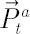denote simple
rates of return for assets on the interval, whereis small enough. By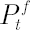we shall designate corresponding simple rate of return of an unobserved factor.

Suppose that the dynamics of the portfolio components satisfies the one-factor model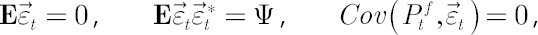wheredenotes vector of betas and vector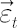contains regression residuals.

[MacKinlay, Pastor; 2000] is that the covariance’s matrix of the residuals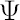is proportional to the identity matrix:The above assumption, basically, is not burdensome if portfolio components belong to the same asset class (big-cap stocks, small-cap stocks, bonds etc.).

With the assumptions made it is possible to establish a connection between a covariance matrixand a vector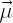, as a result one obtains estimatesand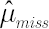. Analytical formulas used in calculation ofandare given in
[Kan, Zhou, 2005].